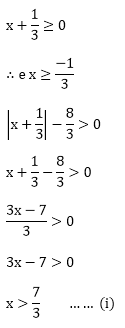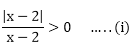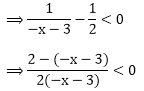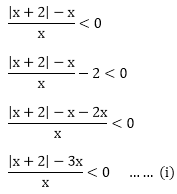#### Thank you for registering.

One of our academic counsellors will contact you within 1 working day.

Click to Chat

1800-5470-145

+91 7353221155

CART 0

• 0
MY CART (5)

Use Coupon: CART20 and get 20% off on all online Study Material

ITEM
DETAILS
MRP
DISCOUNT
FINAL PRICE
Total Price: Rs.

There are no items in this cart.
Continue Shopping

# Chapter 15: Linear Inequations – Exercise 15.3

## Linear Inequations – Exercise – 15.3 – Q.1

Consider the first inequation,Consider the second inequation,## Linear Inequations – Exercise – 15.3 – Q.2

We have,

|4 - x| + 1 - 3 < 0

⟹ |4 - x| - 2 < 0

⟹ 4 - x - 2 < 0

⟹ 2 - x < 0

⟹ - x < -2

⟹ x > 2        ....(ii)

Case II: When |4 - x| < 0

|4 - x| - 2 < 0

⟹ -(4 - x) -2 < 0

⟹ - 4 + x - 2 < 0

⟹ x - 6 < 0

⟹ x < 6               ....... (iii)

Combining (ii) and (iii) we get (2, 6) as the solution set.

## Linear Inequations – Exercise – 15.3 – Q.3Case I: When |3x - 4| ≥ 0⟹ 18x – 24 – 5 ≤ 0

⟹ 18x – 29 ≤ 0

⟹ 18x ≤ 29Case II: When |3x - 4|< 0## Linear Inequations – Exercise – 15.3 – Q.4

We have,Case I: When |x - 2| ≥ 0

x ≥ 2⟹ x – 2 ≥ 0

⟹ x ≥ 2  … (ii)

Case II: When |x - 2| < 0

x < 2⟹ - (x - 2) > 0

⟹ - x + 2 < 0

⟹ - x < - 2

⟹ x > 2        …… (iii)

Combining (ii) and (iii) we get (2, ∞) as the solution set.

## Linear Inequations – Exercise – 15.3 – Q.5

We have,Case I: When |x| ≥ 0 ⟹ x ≥ 0⟹ – x + 5 < 0

⟹ x > 5   ….. (ii)

Case II: When |x|< 0, x < 0⟹ 2 + x + 3 < 0

⟹ x + 5 < 0

⟹ x < - 5          …. (iii)

Combining (ii) and (iii) we get (-∞, -5) ∪ (-3, 3)∪(5, ∞) as the solution set.

## Linear Inequations – Exercise – 15.3 – Q.6

We have,Case I: When |x + 2| ≥ 0

i. e. x ≥ - 2⟹ - 2x + 2 < 0

⟹ - 2x < - 2 and x > 0

⟹ x > 1             …….. (ii)

Case II: |x + 2| < 0

i. e. x < - 2

⟹ - (x + 2) - 3x < 0

⟹ - x – 2 - 3x < 0

⟹ - 4x – 2 < 0

⟹ - 4x < 2

⟹ x > -1/2            …… (iii)

and x < 0

Combining (ii) and (iii) we get (-∞, 0) ∪ (1, ∞) as the solution set.

## Linear Inequations – Exercise – 15.3 – Q.7

We have,Case I: When |2x - 1| ≥ 0

i. e. 2x – 1 ≥ 0

2x ≥ 1

x ≥ 1/2

⟹|2x - 1| - 2x + 2 > 0 and x - 1 > 0

⟹ 2x – 1 - 2x + 2 > 0 and x > 1

⟹ x > 1                 …….. (ii)

Case II: When |2x - 1|< 0

i. e. 2x – 1 < 0

2x < 1

x < 1/2

⟹ - (2x - 1) -2x + 2 > 0 and x < 1

⟹ - 4 + 3 > 0## Linear Inequations – Exercise – 15.3 – Q.8

We have,

|x - 1| + |x - 2| + |x - 3| - 6 ≥ 0   ...... (i)

Case I: |x - 1| ≥ 0

x ≥ 1

⟹ x -1 -(x - 2) - (x - 3) - 6 ≥ 0

⟹ -x + 4 - 6 ≥ 0

⟹ -x ≥ 2

⟹ x ≤ -2

⟹ (-∞,-2]       ..... (ii)

Case II: |x - 2| ≥ 0

x ≥ 2

⟹ x - 1 + x - 2 - (x - 3) - 6 ≥ 0

x - 6 ≥ 0

x ≥ 6

⟹ [6, ∞)     .......  (iii)

Case III: When |x - 3| ≥ 0

x ≥ 3

⟹ x - 1 + x - 2 + x - 3 - 6 ≥ 0

⟹ 3x - 12 ≥ 0

⟹ 3x ≥ 12

⟹ x ≥ 4

⟹ ∴ x ϵ [4, ∞)

also

⟹ |x - 1| < 0

⟹ x < 1

⟹ -(x - 1) - (x - 2) - (x - 3) - 6 ≥ 0

⟹ -3x ≥ 0

⟹ x ≤ 0

⟹ |x - 2| < 0

x < 2

⟹ (x - 1) - (x - 2) - (x - 3) - 6 ≥ 0

⟹ x - 1 - x + 2 - x + 3 - 6 ≥ 0

⟹ -x -2 ≥ 0

⟹ x ≤ -2

⟹ |x - 3| < 0

⟹ x < 3

⟹ (x - 1) + (x - 2) - (x - 3) - 6 ≥ 0

⟹ x - 6 ≥ 0

⟹ x ≥ 6

Combining all cases we get (-∞, 0] ∪ [4, ∞) as the solution set.